## Solution to 1986 Problem 15

 We assume the particles are not interacting and that each particle has an equal probability to be anywhere in the box. Then the probability that a particle is within a volume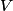$V$ is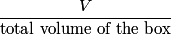\begin{align*}\frac{V}{\mbox{total volume of the box}}\end{align*}So, the probability that a particle is confined to a volume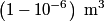$\left(1 - 10^{-6}\right) \;\mathrm{m}^3$ is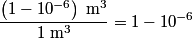\begin{align*}\frac{\left(1 - 10^{-6}\right) \;\mathrm{m}^3}{ 1 \;\mathrm{m}^3} = 1 - 10^{-6}\end{align*}Because we assume that the particles are non-interacting, the probabilities for different particles are independent of one another, and so the probability that all of the particles are confined to a volume of$\left(1 - 10^{-6}\right) \;\mathrm{m}^3$ is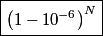\begin{align*}\boxed{\left(1 - 10^{-6} \right)^N}\end{align*}Hence, answer (C) is correct.